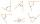# Find the 9

Find the missing angle in the triangle and then name triangle. Angles are: 95, 2x+15, x+3

Result

x =  22.333
A =  95 °
B =  59.666 °
C =  25.333 °

#### Solution:

95+(2x+15)+(x+3)=180

3x = 67

x = 673 ≈ 22.333333

Calculated by our simple equation calculator.Leave us a comment of example and its solution (i.e. if it is still somewhat unclear...):

Showing 0 comments:Be the first to comment!#### To solve this example are needed these knowledge from mathematics:

Do you have a linear equation or system of equations and looking for its solution? Or do you have quadratic equation? See also our trigonometric triangle calculator.

## Next similar examples:

1. SlopeFind the slope of the line: x=t and y=1+t.
2. Triangle anglesIn a triangle ABC the interior angle at the vertex C is twice as the internal angle at the point A. Outer angle at the point B measured 117 degrees. How big is the outer angle at the vertex A?
3. Largest angle of the triangleWhat is the largest angle of the triangle if the second angle is 10° greater than twice the first and the third is 30° smaller than the second?
4. Supplementary anglesOne of the supplementary angles are three times larger than the other. What size is larger of supplementary angles?
5. Center traverseIt is true that the middle traverse bisects the triangle?
6. Fifth of the numberThe fifth of the number is by 24 less than that number. What is the number?
7. CompetitorsIn the first round of slalom fell 15% of all competitors and in the second round another 10 racers. Together, 40% of all competitors fell. What was the total number of competitors?
8. Football seasonDalibor and Adam together scored 97 goals in the season. Adam scored 9 goals more than Dalibor. How many goals scored each?
9. Reciprocal equationDetermine the root of the equation: 9/x-7/x=1
10. Family parcelsIn father will he divided the land so that the older son had three bigger part than younger son. Later elder son gave 2.5 ha field to younger and they had both the same. Determine the area of family parcel.
11. SummerjobThree students participated in the summerjob. Altogether they earn 1780, -. Peter got a third less than John and Paul got 100,- more than Peter. How much got every one of them?
12. Perctentages35% of what number is 35?
13. ClassroomThere are eighty more girls in the class than boys. Boys are 40 percent and girls are 60 percent. How many are boys and how many girls?
14. BlackberriesDaniel, Jolana and Stano collected together 34 blackberries. Daniel collected 8 blackberries more than Jolana, Jolana 4 more than Stano. Determine the number blackberries each collected .
15. Mom and daughterMother is 39 years old. Her daughter is 15 years. For many years will mother be four times older than the daughter?
16. Dining roomThe dining room has 11 tables (six and eight seats). In total there are 78 seats in the dining room. How many are six-and eight-seat tables?
17. PupilsThere are 350 girls in the school, and the other 30% of the total number of pupils are boys. How many pupils does the school have?